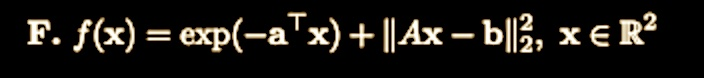### Composite function convexity

Could someone please explain how to show convexity, strong convexity, strict convexity of the composite function:Top comment

Since $$\nabla^2 f(x) = \exp(-a^Tx) aa^T + 2A^TA$$, we have that $$|| \nabla^2 f(x) || > 2||A^TA||$$ for any $$x \in \mathbb{R}^2$$, which shows strong convexity, and strong convexity implies strict convexity and convexity.

Note that it would not be true if $$||A^TA||_2 = 0$$, but we can show that $$A^TA = I_2$$.

How does that show strong convexity? Could you point to a lemma maybe? Thanks!

Lemma 4.9 on page 81 : if $$f(x)$$ is twice differentiable then you can take the Hessian of the left and right hand side, then combining with the convexity conditions it becomes :
$$\nabla^2 f(x) - \mu \succcurlyeq 0$$

So for the question above you have that the largest eigenvalue of $$I_2$$ is 1, and thus $$\mu = 2$$.

Thanks for the answer, but I still don't see why you can just ignore the fact that a is in R^2 and hence it can be positive or negative. To my mind that means that the hessian can for certain a be unbounded.

Page 1 of 1